## Pages

### NPOI with Excel Table and dynamic Chart

A reader of the blog called Zip wrote a comment on the post Creating Excel spreadsheets .XLS and .XLSX in C#.

This is an excerpt from Zip’s comment:

if I add rows using NPOI in C#, rows added under the table won't be automatically included in the table, and my chart is not updated the way I would like it to be.
How can I work around this problem?

I tried to simulate the problem with a simple spreadsheet and I was getting the same problem stated by Zip, that is, if I added one row just beneath the last row in the table, such added row wasn’t included in Excel’s data table and consequently the chart bound to the table wasn’t updated to reflect the new data.

To workaround this problem, let’s consider the following spreadsheet shown in Figure 1: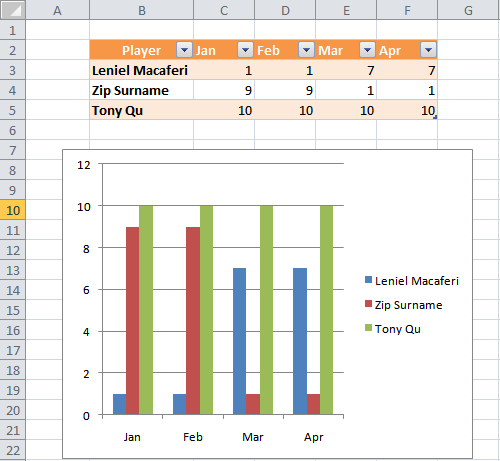Figure 1 - NPOI with Excel Table and dynamic Chart

As you see we have a simple Excel data table with a players column that represents the name arguments of the chart, 4 columns for the months that form the category labels arguments (X axis) and the values arguments for the months going from Jan through Apr (Y axis).

Using NPOI to insert a new row in the table shown above we do the following:

```// Creating a new row... 0 is the first row for NPOI.
HSSFRow row = sheet.CreateRow(5); // Row 6 in Excel```
```// Creating new cells in the row... 0 is the first column for NPOI.
row.CreateCell(2).SetCellValue(4); // Column C
row.CreateCell(3).SetCellValue(3); // Column D
row.CreateCell(4).SetCellValue(2); // Column E
row.CreateCell(5).SetCellValue(1); // Column F ```

The result is shown in Figure 2: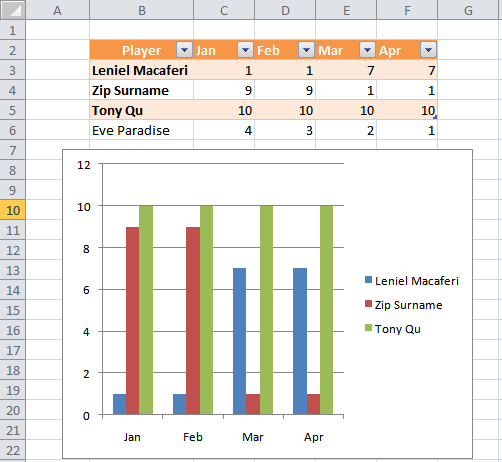Figure 2 - NPOI with Excel Table and dynamic Chart - Adding a new row

Figure 2 shows us the problem stated by Zip in his comment. The new row we just added wasn’t included in the table. The chart that is linked to the table won’t update because it isn’t aware of the new row.

How to workaround this problem? That’s the question!

After playing with this case for 4 hours I’ve found a way of doing what Zip asks for.

Here’s how I did it:

Expand your Excel data table to row 10. I expanded only 4 rows just to show you how to workaround NPOI’s current limitation.

To expand your table, click in the minuscule handle in the lower-right corner of the cell occupying the lower-right corner of the table. This handle gives you a way to expand the table. Usually, it’s easier just to add data and let Excel expand the table - what doesn’t work with NPOI. But if you want to add several new rows or columns all at once, the handle is a good way to do it.

Figure 3 shows how the above spreadsheet looks like when the table is expanded to row 10: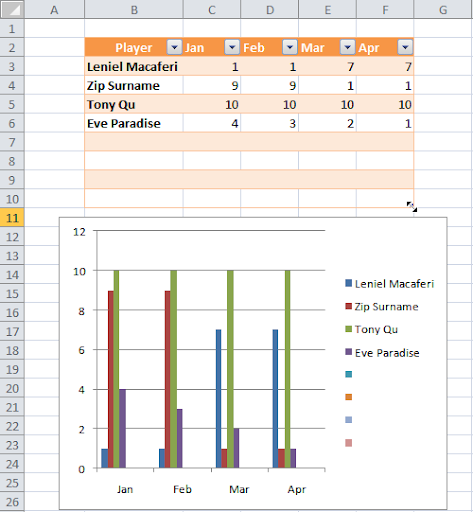Figure 3 - NPOI with Excel Table and dynamic Chart - Expanding the Table

We can see that row 6 added using NPOI is now part of the table because we expanded the table. The chart now shows the new data but we got a new problem: the chart shows empty (blank series) that are the reflection of the the empty rows we have on the data table - take a look at the chart’s legend for example and you’ll see squares that represent nothing.

How to get over this? Well, we just need to filter the data in the table as shown in

Figure 4: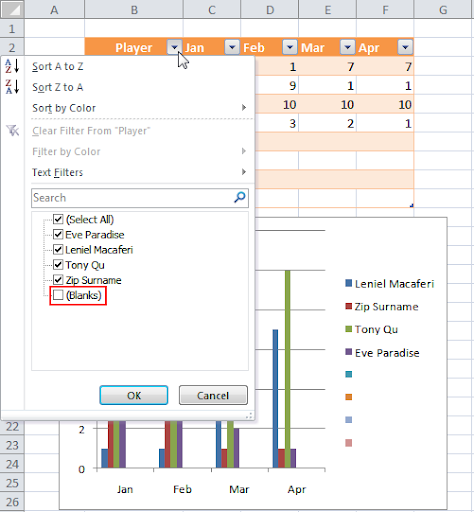Figure 4 - NPOI with Excel Table and dynamic Chart - Filtering Data (blank series)

Filter out players removing the blank rows by unchecking (Blanks) circled in red in Figure 4. Doing so the chart will reflect the change showing only the filtered data as you see in Figure 5: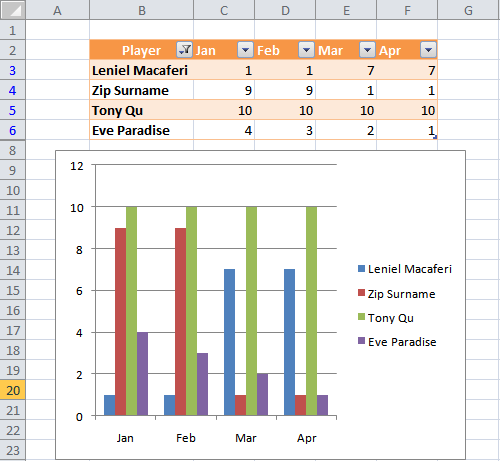Figure 5 - NPOI with Excel Table and dynamic Chart - Filtered Data (no empty rows)

Now we have an Excel data table that is filtered (take a look at the funnel symbol) in the Player column. Other difference is that the rows that contain data are marked in blue. Although we have only 4 rows of data being displayed, our table has indeed 8 rows of data because we expanded it. The other 4 rows are hidden because they were filtered for not having any data yet.

Positioning the mouse cursor within the Excel data table, I’ll add a Total Row (option circled in red) in the table so that I can summarize data the way I want for each column as shown in Figure 6: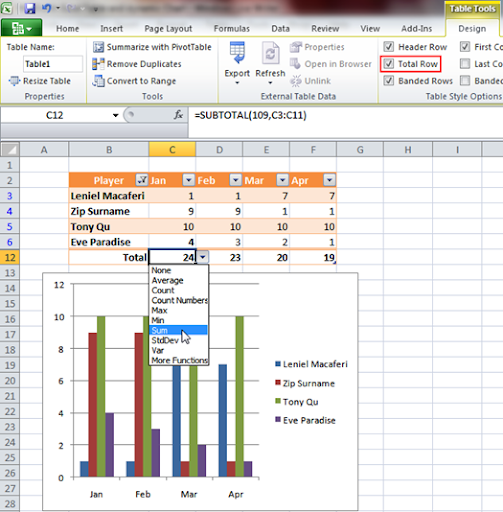Figure 6 - NPOI with Excel Table and dynamic Chart - Adding Total Row

With this Excel template spreadsheet we can now use NPOI to fill our sheet with more 4 rows of data. Let’s do it. This is the code I used:

```HSSFRow row7 = sheet.CreateRow(6);

row7.CreateCell(1).SetCellValue("David Goliath");
row7.CreateCell(2).SetCellValue(7);
row7.CreateCell(3).SetCellValue(7);
row7.CreateCell(4).SetCellValue(7);
row7.CreateCell(5).SetCellValue(7);

HSSFRow row8 = sheet.CreateRow(7);

row8.CreateCell(2).SetCellValue("Moses of Egypt");
row8.CreateCell(3).SetCellValue(8);
row8.CreateCell(4).SetCellValue(8);
row8.CreateCell(5).SetCellValue(8);
row8.CreateCell(6).SetCellValue(8);

HSSFRow row9 = sheet.CreateRow(8);

row9.CreateCell(1).SetCellValue("David Shepherd");
row9.CreateCell(2).SetCellValue(9);
row9.CreateCell(3).SetCellValue(9);
row9.CreateCell(4).SetCellValue(9);
row9.CreateCell(5).SetCellValue(9);

HSSFRow row10 = sheet.CreateRow(9);

row10.CreateCell(2).SetCellValue("Jesus of Nazareth");
row10.CreateCell(3).SetCellValue(10);
row10.CreateCell(4).SetCellValue(10);
row10.CreateCell(5).SetCellValue(10);
row10.CreateCell(6).SetCellValue(10);```
`// Forcing formula recalculation so that the Total Row gets updatedsheet.ForceFormulaRecalculation = true;`

After filling the spreadsheet we get the result shown in Figure 7: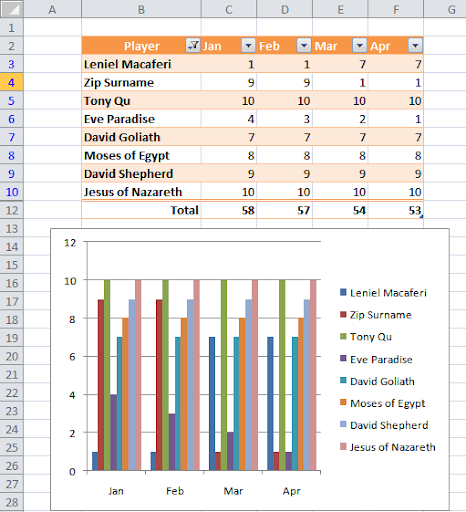Figure 7 - NPOI with Excel Table and dynamic Chart - Chart updated automatically/dynamically

This is the workaround! :o)

The rows added with NPOI now are part of the table and are shown in the chart.

As a last hint: remember to expand your Excel data table to the number of rows you think your spreadsheet will store so that the rows added with NPOI get included in the table and the chart gets updated.

Again this is a good proof of what free software as is the case of NPOI can make for us. Even when dealing with more elaborated concepts as is the case of Excel tables and charts NPOI makes it easy to get the job done.

I wish that the next version of NPOI does what Zip wants automatically, that is, recognize rows added under the last row of an Excel table. At least we could have a parameter to let the user define if s/he wants the row to make part of the table or not.

Hope you enjoy this post.

Visual Studio 2008 C# ASP.NET MVC Web Application
You can get the Microsoft Visual Studio Project at: